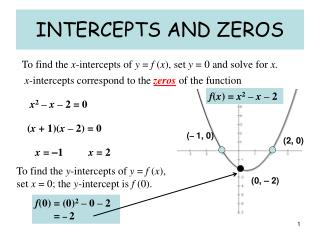Download PresentationINTERCEPTS AND ZEROS

# INTERCEPTS AND ZEROS - PowerPoint PPT Presentation

INTERCEPTS AND ZEROS. To find the x -intercepts of y = f ( x ), set y = 0 and solve for x. x -intercepts correspond to the zeros of the function. f ( x ) = x 2 – x – 2. x 2 – x – 2 = 0. ( x + 1)( x – 2) = 0. ( – 1, 0). (2, 0). x = – 1. x = 2.I am the owner, or an agent authorized to act on behalf of the owner, of the copyrighted work described.
Download Presentation## INTERCEPTS AND ZEROS

An Image/Link below is provided (as is) to download presentation

Download Policy: Content on the Website is provided to you AS IS for your information and personal use and may not be sold / licensed / shared on other websites without getting consent from its author.While downloading, if for some reason you are not able to download a presentation, the publisher may have deleted the file from their server.

- - - - - - - - - - - - - - - - - - - - - - - - - - E N D - - - - - - - - - - - - - - - - - - - - - - - - - -
Presentation Transcript
1. INTERCEPTS AND ZEROS To find the x-intercepts of y = f (x), set y = 0 and solve for x. x-intercepts correspond to the zeros of the function f(x) = x2 – x – 2 x2 – x – 2 = 0 (x + 1)(x – 2) = 0 (–1, 0) (2, 0) x = –1 x = 2 To find the y-intercepts of y = f (x), set x = 0; the y-intercept is f (0). (0, – 2) f(0) = (0)2 – 0 – 2 = – 2

2. EXAMPLE 1 f(x) = 3x2 + 7x – 6 x-intercepts (Set y = 0) y-intercept (Set x = 0) 3x2 + 7x – 6 = 0 f(0) = 3(0)2 + 7(0) – 6 3x2 + 9x – 2x – 6 = 0 f(0) = – 6 3x (x + 3) – 2(x + 3) = 0 (3x – 2)(x + 3) = 0 (– 3, 0) (2/3, 0) x = 2/3 x = -3 (0, -6)

3. (0, 1) EXAMPLE 2 f(x) = 2 sin x, -p<x<p 1 y x-intercepts (Set y = 0) y-intercept (Set x = 0) q (1, 0) x (– 1, 0) (0, – 1) Also

4. 2 1 2 EXAMPLE 3 f(x) = 2cos x – 1, 0 <x< 2p x-intercepts (Set y = 0) y-intercept (Set x = 0)

5. Symmetry (–x , y) ( x , y) An even function satisfies f (– x) = f ( x ). The graph of an even function is symmetric about the y-axis

6. EXAMPLE 4 f(x) = 9 – x2 Show that this is an even function. It is symmetrical to the y-axis f(–x) = 9 – (–x)2 f(–x) =9 – x2 (– 2, 5) (2, 5) (– 3, 0) (3, 0)

7. EXAMPLE 5 f(x) = x4 – 4x2 Show that this is an even function. It is symmetrical to the y-axis f(–x) = (–x)4 – 4(–x)2 f(–x) =x4 – 4x2 (– 2, 0) (2, 0) (– 1, – 3) (1, – 3)

8. (x, y) (-x, -y) An odd function satisfies f (– x) = – f ( x ) The graph of an odd function is symmetric about the origin.

9. EXAMPLE 6 f(x) = x3– 9x Show that this is an odd function. It is symmetrical to the origin An odd function satisfies f (– x) = – f ( x ) f(-x) =(-x)3 – 9(-x) – f(x) =– (x3 – 9x ) f(-x) = – x3 + 9x –f(x) = – x3 + 9x (– 2, 10) (2, – 10)

10. EXAMPLE 7 Determine if the function is even, odd, or neither. Find –f(x): Find f(-x): = Since f(x) ≠ f(– x), the function is noteven and not symmetric about the y-axis. Since f(– x) = – f(x) the function is odd and symmetric about the origin

11. EXAMPLE 8 y = 4x2 – x Determine if the function is even, odd, or neither. Find –f(x): Find f(– x): ≠ Since f(x) ≠ f(–x), the function is noteven and not symmetric about the y-axis. Since f(–x) ≠– f(x) the function is notodd and notsymmetric about the origin